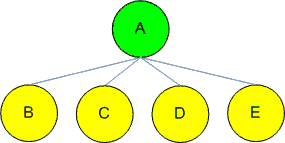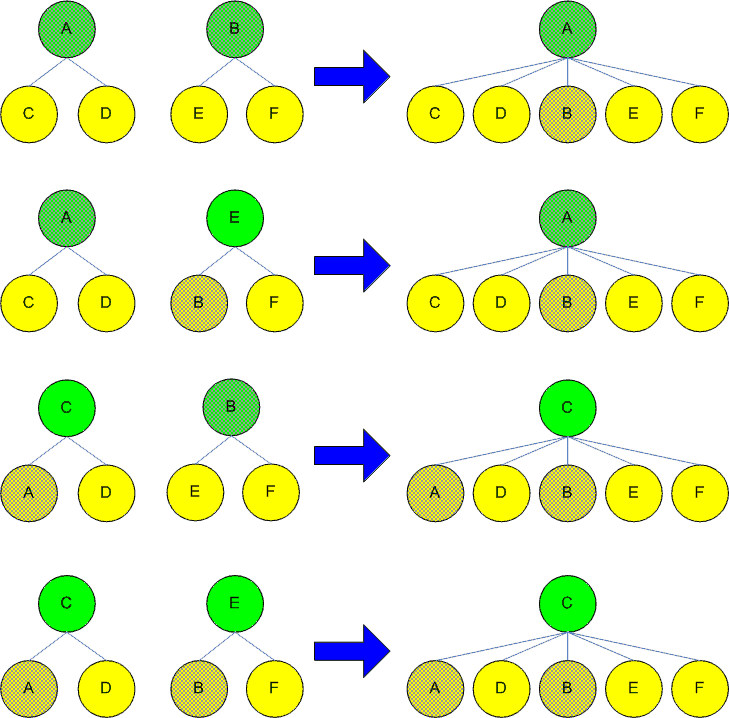A two level tree is a simple tree data structure. Unlike in a typical tree where the tree depths could be arbitrary, a two level tree has only two levels as its name suggests. Two level tree is also equivalent to a star.The following graph illustrates what a two level tree looks like:A two level tree

There is no internal nodes in a two level tree. A two level tree has only a root node and a set of leaf nodes.

There are two types basic of operations we can perform on a two level tree: we can Add a two level tree to another two level tree and thus form a new two level tree or we can remove a node or a set of nodes from a two level tree.

To add a two level tree to another two level tree, we need to ensure that the combined tree remains a two level tree. when we say adding a tree, it means adding the "whole tree". But in practice, we really do not care which part of the tree we refer to. For instance, the above tree can be referred to by A (the root node), or by any of the four children (e.g. C). Thus there are four scenarios when adding two trees together, depending on how the tree is referenced. The following graph illustrate this:Merging Two Two Level Trees

The above four different scenarios depend on how the tree is referenced (the shaded letters). We could mandate that a tree referenced by its root node and thus simplify the merging algorithm, but you will see in a later post that this approach adds more flexibility. The following code (C#) shows how the above steps are accomplished:

namespace TwoLevelTree
{
public class TreeNode
{
private string _ID = null;
private TreeNode _parent = null;
private List<TreeNode> _children = null;

public string ID
{
get { return _ID; }
set { _ID = value; }
}

public TreeNode Parent
{
get { return _parent; }
set { _parent = value; }
}

public List<TreeNode> Children
{
get { return _children; }
set { _children = value; }
}

public TreeNode(string id)
{
_ID = id;
}

{
if (this.Parent == null)
{
if (this.Children == null)
{
this.Children = new List<TreeNode>();
}

node.Parent = this;

if (node.Children != null)
{
foreach (TreeNode n in node.Children)
{
n.Parent = this;
}

node.Children = null;
}
}
else
{
node.Parent = this.Parent;

if (node.Children != null)
{
foreach (TreeNode n in node.Children)
{
n.Parent = this.Parent;
}

node.Children = null;
}
}

return this;
}

public TreeNode Remove(TreeNode node)
{
if (node.Parent != null)
{
this.Children.Remove(node);
}
else
{

this.ID = _children.ID;
_children.RemoveAt(0);

foreach (TreeNode n in _children)
{
n.Parent = this;
}

}

return this;
}

public override string ToString()
{
StringBuilder sb = new StringBuilder();

if (_parent == null)
{
sb.Append(string.Format("({0});", _ID));

if (_children != null)
{
foreach (TreeNode n in _children)
{
sb.Append(n.ToString());
}
}
}
else
{
sb.Append(string.Format("{0};", _ID));

if (_children != null)
{
foreach (TreeNode n in _children)
{
sb.Append(n.ToString());
}
}
}

return sb.ToString();
}
}

class Program
{
static void Main(string[] args)
{
TreeNode n1 = new TreeNode("t1");
TreeNode n2 = new TreeNode("t2");
TreeNode n3 = new TreeNode("t3");

TreeNode n4 = new TreeNode("t4");
TreeNode n5 = new TreeNode("t5");
TreeNode n6 = new TreeNode("t6");

Console.WriteLine(t1);

Console.WriteLine(t2);

Console.WriteLine(t3);

TreeNode t4 = t3.Remove(n4);
Console.WriteLine(t4);

TreeNode t5 = t4.Remove(n1);
Console.WriteLine(t5);